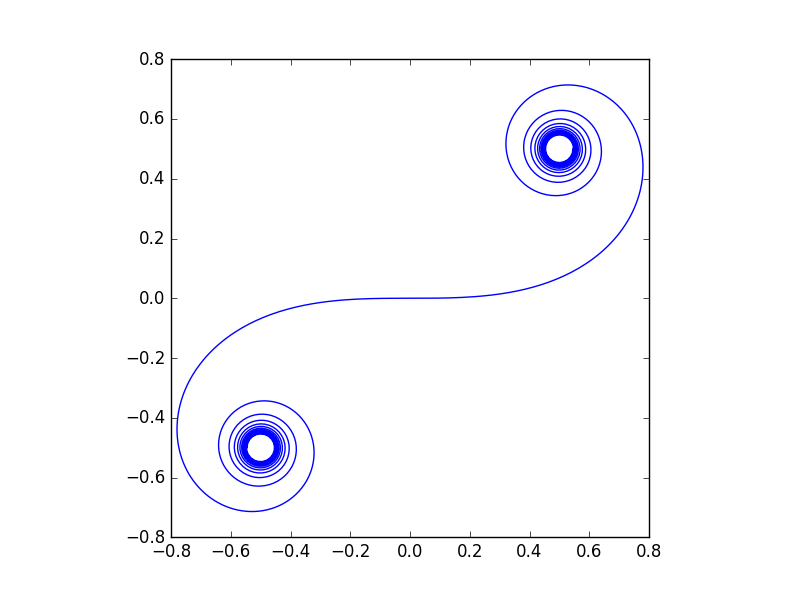# Cornu's Spiral

### Some of us at DZone develop scientific/engineering/research code. And to people like us, an elegant and simple mathematical function can be a powerful tool. We might not use it today but it's nice to have in the toolbox!

· Big Data Zone · Opinion
Save
2.68K Views

cornu’s spiral is the curve parameterized by:where c and s are the fresnel functions, sometimes called the fresnel cosine integral and fresnel sine integral. here’s a plot of the spiral.both fresnel functions approach ½ as t → ∞ and so the curve slowly spirals toward (½, ½) in the first quadrant. and by symmetry, because both functions are odd, the curve spirals toward (-½, -½) in the third quadrant.

here’s the python code used to make the plot:

    from scipy.special import fresnel
from scipy import linspace
import matplotlib.pyplot as plt

t = linspace(-7, 7, 1000)
y, x = fresnel(t)

plt.plot(x, y)
plt.axes().set_aspect("equal")
plt.show()


the scipy function  fresnel  returns both fresnel functions at the same time. it returns them in the order ( s , c ) so the code reverses the order of these to match the cornu curve.

one interesting feature of cornu’s spiral is that its curvature increases linearly with time. this is easy to verify: because of the fundamental theorem of calculus, the fresnel functions reduce to sines and cosines when you take derivatives, and you can show that the curvature at time t equals π t .

cornu’s spiral was actually discovered by euler. cornu was an engineer who independently discovered the curve much later. perhaps because cornu used the curve in applications, his name is more commonly associated with the curve. at least i’ve more often seen it named after cornu. this is an example of stigler’s law that things are usually not named after the first person to discover them.

Topics:
algorithm, mathematical programming, python

Published at DZone with permission of John Cook, DZone MVB.

Opinions expressed by DZone contributors are their own.